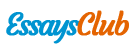# Stepped Solar Still

Autor:   •  October 15, 2018  •  2,457 Words (10 Pages)  •  151 Views

Page 1 of 10

...

Here, T1 is the inlet temperature to the first coaxial pipe and its value is 25⁰C.

T2 is the exit temperature of the first coaxial pipe and its value is determined by solving above equation.

Energy balance of the coaxial pipe from Fig. 3.

(2) [pic 9]

The net amount of heat per unit time which increase temperature of saline water across one pipe is given by:

(1)[pic 10]

This heat can be calculated from energy balance for the saline water from Fig. 4.

c-sw[pic 11][pic 12]

r(sw-g)c(sw-g) evap (3)[pic 13][pic 14][pic 15]

Asw represents the opening area through which water evaporates to the glass.

Asw = L * (thickness of opening)

Heat transfer between coaxial pipe and water is convection because water is flowing so there is movement of particles and it is calculated as follows:

c-sw c - T1) (4) [pic 16][pic 17]

The heat losses by convection through the base and sides to the ground and surrounding, Qlost given as

(5) [pic 18]

The convective heat transfer between saline water and the glass cover, Qc(sw–g), is given by

(6) [pic 19]

Where =[pic 20]

(7)[pic 21]

[pic 22]

[pic 23]

The radiation heat transfer from the saline water to glass cover, Qr(sw-g), is given by:

(8) [pic 24]

Also, the radiation shape factor as a function of the glass cover inclination angle is correlated with the maximum deviation of 0.06% by the following

1.68*10-44 (9) where can be found from Fig. 5. using [pic 25][pic 26][pic 27][pic 28]

(10) [pic 29]

Fig. 5.

θ calculation

[pic 30]

The evaporative heat transfer between saline water and the glass is given by

(11) [pic 31]

• Now we know all variables we can easily find Qnet from equation (3) and then use eq(1) to find T2 .This T2 becomes start point of next pipe ie for second pipe T1(second pipe ) = T2 (of first pipe)

Energy balance for glass cover using

Fig. 6.

(12)[pic 32]

For stepped solar still, the radiative heat transfer between the glass and the sky is given by

(13) [pic 33]

For stepped solar still, the convective heat transfer between the glass and the sky is given by

(14)[pic 34]

(15)[pic 35]

The daily efficiency, can be obtained by the sum of hourly condensate

production mp, multiplied by the latent heat Lw at average water temperature Tw, hence the results was divided by the

average solar radiation I(t) over the whole absorber area A

(16)[pic 36]

Tw21.5863*10-5 Tw2 (17) [pic 37][pic 38]

Solar still productivity is given by

(18)[pic 39]

The percentage distillate productivity of the solar still can be calculated as

= 9.008+(0.194*)+(1.465*10-9*5)+(8.652*10-8**I2) (0.004*I) (0.00016**I)(5.07*10-10*I2*2) (19)[pic 40][pic 41][pic 42][pic 43][pic 44][pic 45][pic 46][pic 47][pic 48]

Item

Area(m2)

[pic 49]

α

Glass

0.90

0.85

0.05

Coaxial pipe (Copper)

0.22

0.88

0.50

Saline water

0.0095

0.96

0.05

Table. 2. Physical and operating parameters used in the theoretical calculation.

3. Results and discussion

Increasing the number of coaxial pipes increases the temperature of saline water as more area is available for heat transfer. This can be verified by observing Fig. 6. where increasing the no of coaxial pipes increases surface temperature of saline water. As it can be seen from the graph that water in coaxial pipe solar still has a higher temperature as compared to simple solar still and stepped solar still.

For conventional solar still temperature of saline water increases only at one stage while for stepped solar still temperature increases at each step as there is more contact of water with heated surface.

Comparing stepped solar still with coaxial pipe, the final temperature of our model is higher than the stepped solar still because there is more surface area of cylindrical pipes in contact with water at each step as compared to a flat surface.

[pic 50]

Fig. 6. Temperature variation with different solar still configuration. (NOTE: no of pipes for stepped and conventional solar still refers to the no of steps and increase in length of exposed area respectively)

[pic

...